# Hockey stick identity: How does it work if it starts at the left and not at the right?

• Module 3 Week 2 Day 6 Your Turn Part 2

I came across this identity again, and I had forgotten how the hockey stick behaves if it starts at the left. How does it work if it starts at the left and not at the right?

• @The-Blade-Dancer Good question! It seems like the left-hand-starting hockey stick might be a different case from the right-hand-starting hockey stick... It's really the same case! It sometimes seems like there's a lot to remember. ... How about trying to not remember everything? (I'm being a little facetious.) You only have to remember a little bit:

1.) The binomial coefficients along the very left and right edges are always equal to \(1.\)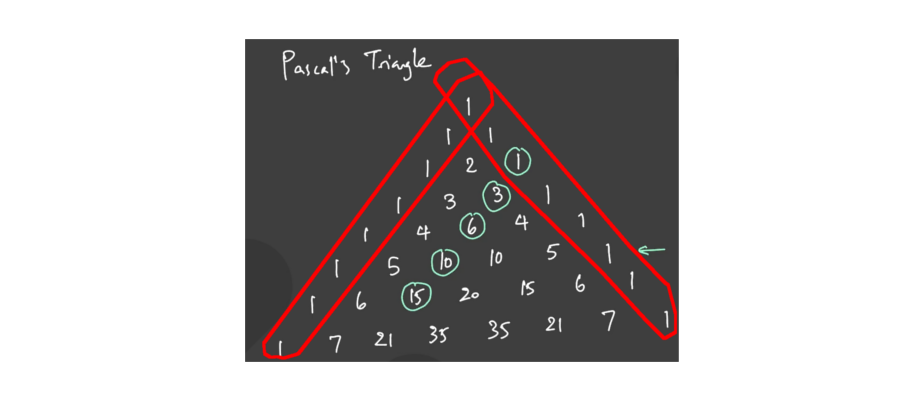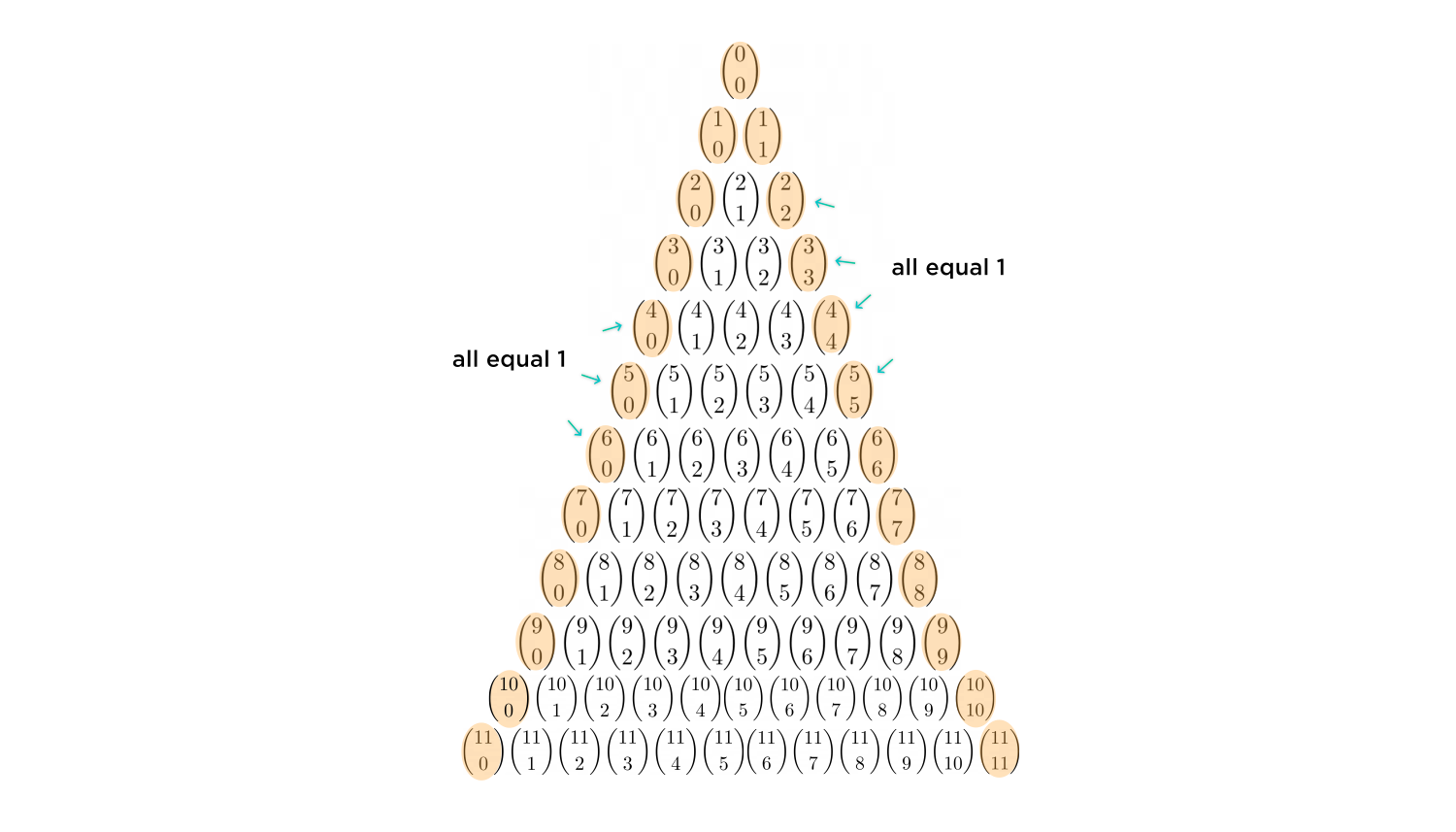2.) To get the value of any number in Pascal's Triangle (or binomial coefficient), you just add up the two numbers directly above it. Pictured below: \( \textcolor{blue}{\binom{6}{1} + \binom{6}{2} = \binom{7}{2}} \)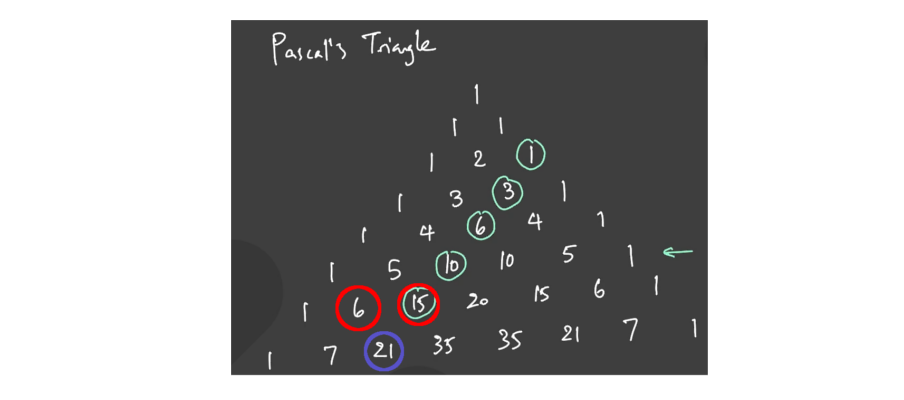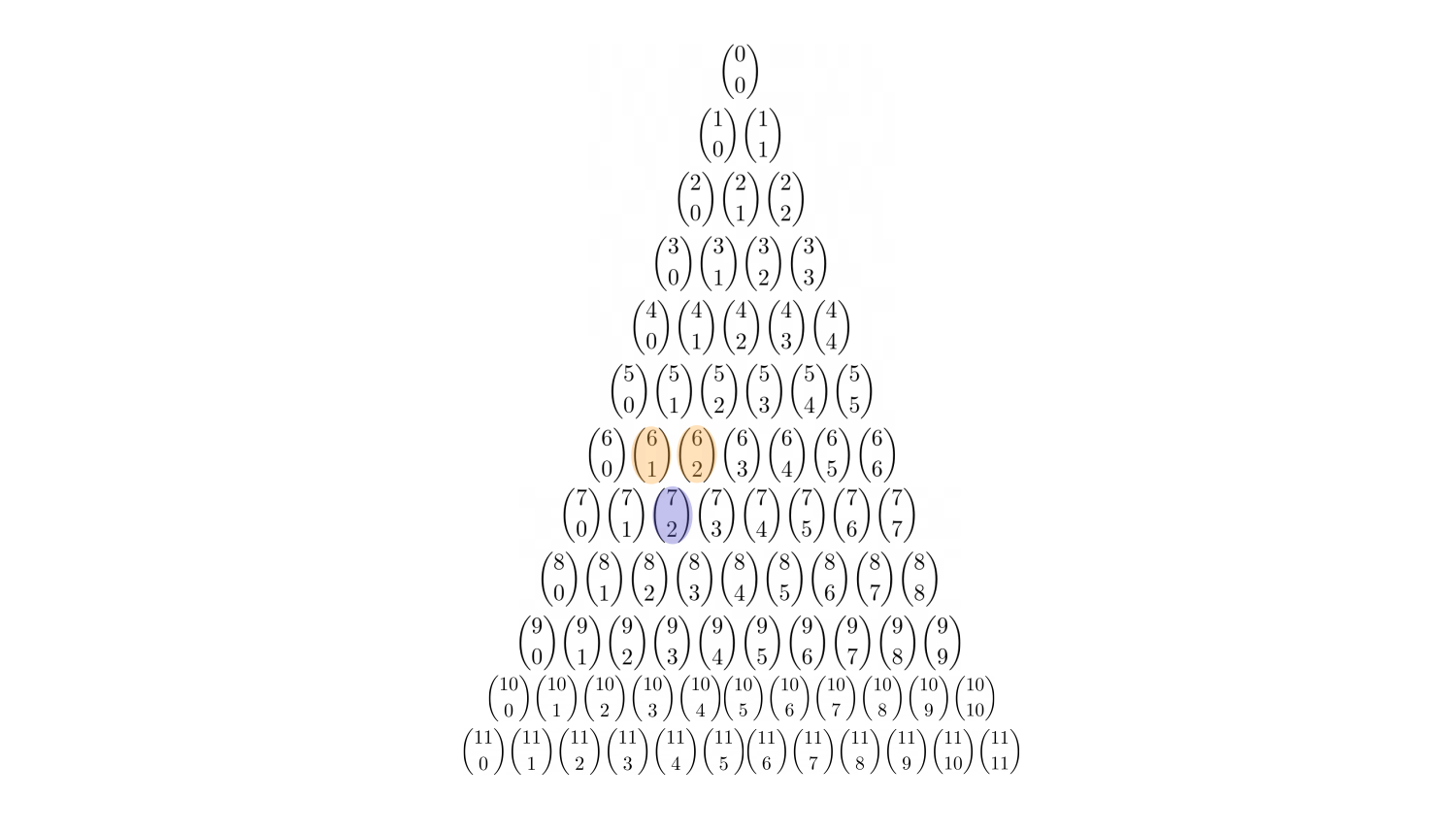In general, \$\$ \binom{n}{m} + \binom{n}{m+1} = \binom{n+1}{m+1} \$\$

These two rules are all you need in order to derive the Hockey Stick Identity, and we can apply them to the left side of Pascal's Triangle as well.

### General Idea:

#### Start a "domino effect," wherein a side-by-side pair of binomial coefficients gets eliminated and is replaced by the coefficient under it, and so on, until there is only one pair of coefficients left.

1.) Let's use an example: \( \binom{4}{0} + \binom{5}{1} + \binom{6}{2} + \binom{7}{3} + \binom{8}{4} + \binom{9}{5} + \binom{10}{6}.\)

We don't have a side-by-side pair yet. That's okay. Start with the binomial coefficient on the very left edge. Since all edge numbers are equal, we can switch this edge number, \( \binom{4}{0},\) with the one below it, \(\binom{5}{0},\) which is now side-by-side with the next coefficient, \(\binom{5}{1}.\)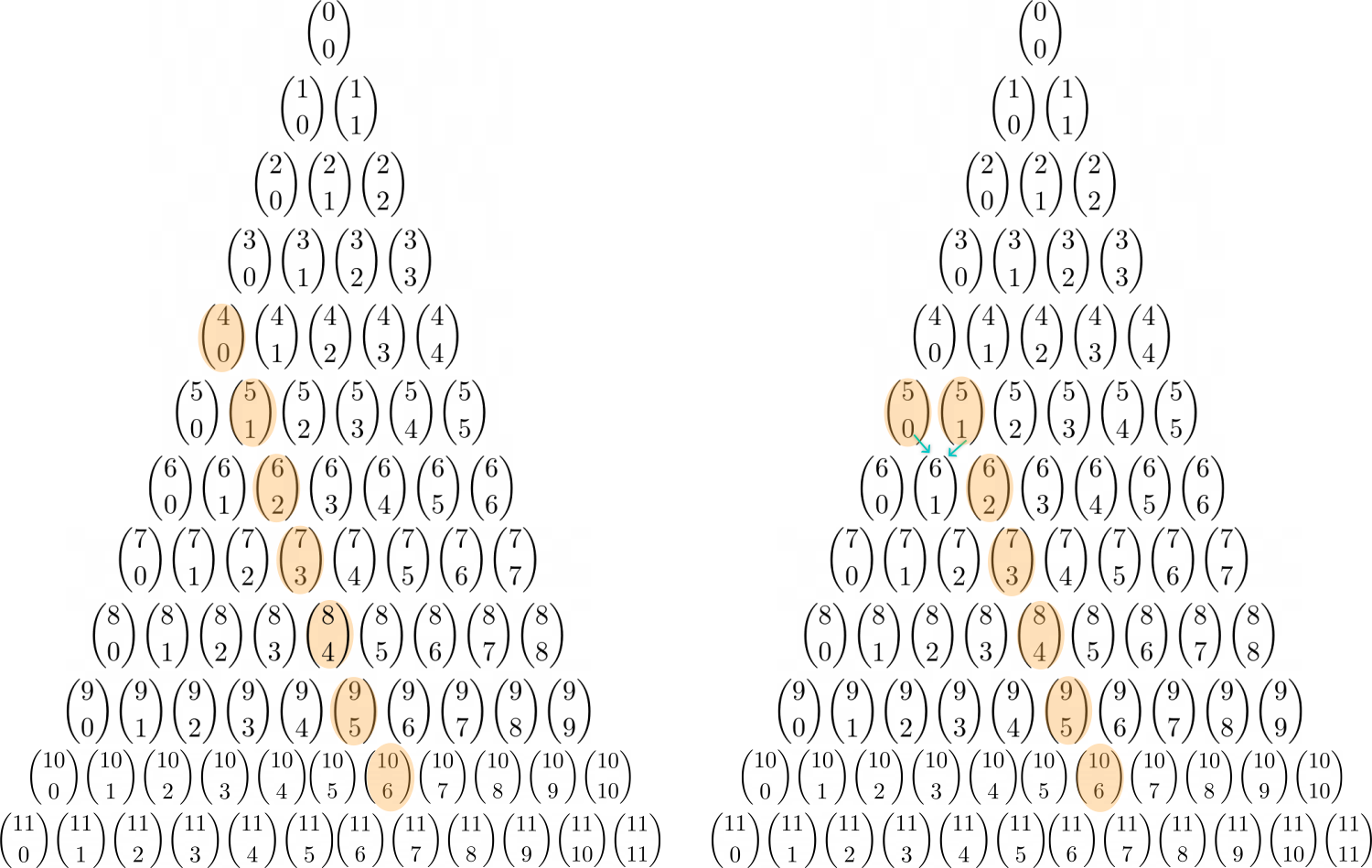Now use Rule 2 to eliminate the \(\binom{5}{0} \) and \(\binom{5}{1}\) and turn them into \(\binom{6}{1}.\)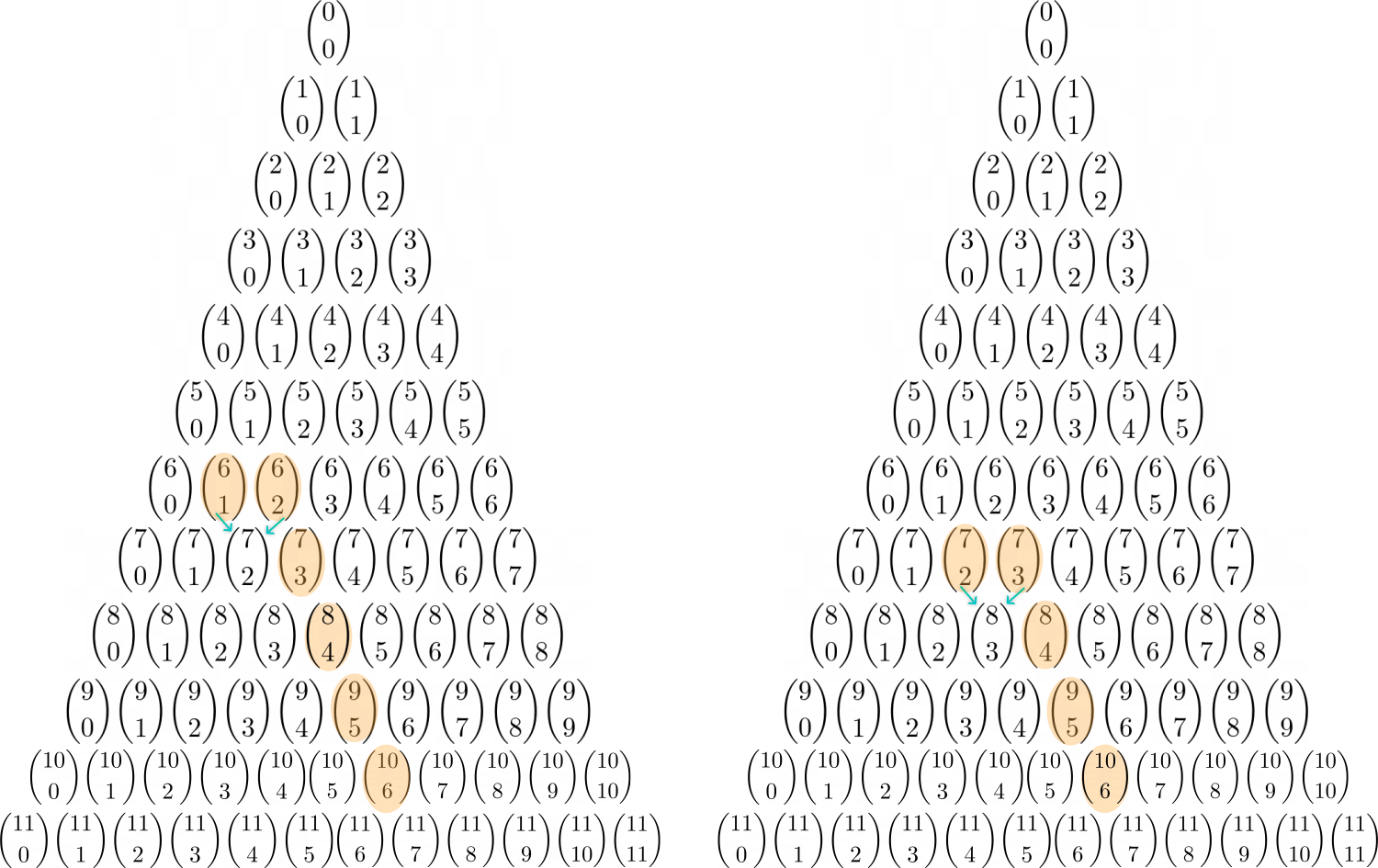We get another side-by-side pair: \( \binom{6}{1}\) and \( \binom{6}{2},\) which, using Rule 2, gets obliterated and replaced by \(\binom{7}{2}.\)

Doing this again, the side-by-side pair \( \binom{7}{2}\) and \( \binom{7}{3}\) turns into \(\binom{8}{3}.\)

We comfortably have only a few chooses left to calculate. But wait, we can do the trick a few more times!

The side-by-side pair \( \binom{8}{3}\) and \( \binom{8}{4}\) gets replaced by \(\binom{9}{4},\) and the side-by-side pair \( \binom{9}{4}\) and \( \binom{9}{5}\) gets replaced by \(\binom{10}{5}.\)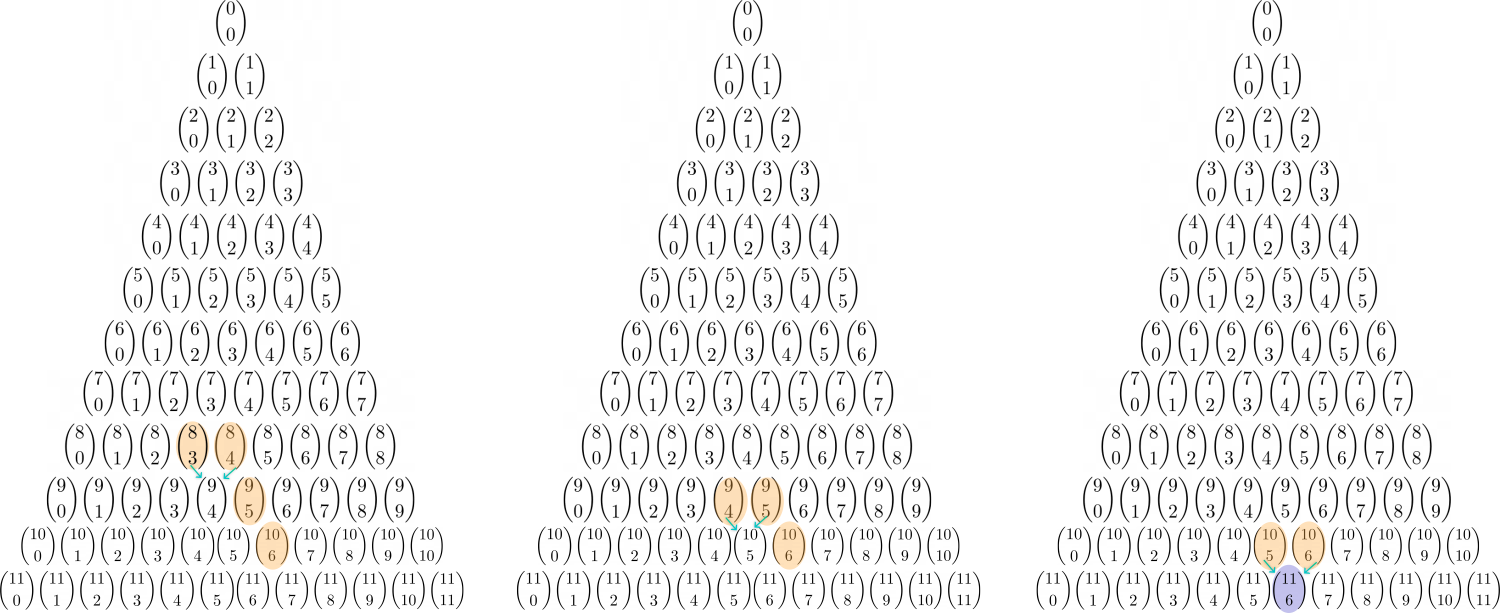At the every end, we only have two binomial coefficients left:

\$\$ \binom{10}{5} + \binom{10}{6},\$\$

which, using Rule 2 again, equals

\$\$ \binom{11}{6}.\$\$

so, referring back to the beginning, we simplified our original expression like this:

\$\$ \textcolor{blue}{\binom{4}{0} + \binom{5}{1} + \binom{6}{2} + \binom{7}{3} + \binom{8}{4} + \binom{9}{5} + \binom{10}{6} = \binom{11}{6}.}\$\$

Also, due to the nice symmetry of Pascal's Triangle, you can just reflect the left hockey stick so it becomes a right hockey stick.• @RZ923 Wow, that is such a cool and simple explanation!!